# Triangle

The essential made for every moment of your week—features transformable and adjustable straps for weightless support with no digging or tugging.

## Filters (0)

• Best Seller
• XS
• XS
• XS
• XS
• XS
• XS
• XS
• XS
• XS
• XS
• S
• S
• S
• S
• S
• S
• S
• S
• S
• S
• M
• M
• M
• M
• M
• M
• M
• M
• M
• M
• L
• L
• L
• L
• L
• L
• L
• L
• L
• L
• XL
• XL
• XL
• XL
• XL
• XL
• XL
• XL
• XL
• XL
• 2XL
• 2XL
• 2XL
• 2XL
• 2XL
• 2XL
• 2XL
• 2XL
• 2XL
• 2XL
• 3XL
• 3XL
• 3XL
• 3XL
• 3XL
• 3XL
• 3XL
• 3XL
• 3XL
• 3XL
• 1+
• 1+
• 1+
• 1+
• 1+
• 1+
• 1+
• 1+
• 1+
• 1+
• 2+
• 2+
• 2+
• 2+
• 2+
• 2+
• 2+
• 2+
• 2+
• 2+
• 3+
• 3+
• 3+
• 3+
• 3+
• 3+
• 3+
• 3+
• 3+
• 3+

Ultra-Soft Re:Play

1680

### Triangle Bralette

\$42–\$48

\$29.40–\$33.60

••••••••••• Best Seller
• XS
• XS
• XS
• XS
• XS
• XS
• XS
• XS
• XS
• XS
• S
• S
• S
• S
• S
• S
• S
• S
• S
• S
• M
• M
• M
• M
• M
• M
• M
• M
• M
• M
• L
• L
• L
• L
• L
• L
• L
• L
• L
• L
• XL
• XL
• XL
• XL
• XL
• XL
• XL
• XL
• XL
• XL
• 2XL
• 2XL
• 2XL
• 2XL
• 2XL
• 2XL
• 2XL
• 2XL
• 2XL
• 2XL
• 3XL
• 3XL
• 3XL
• 3XL
• 3XL
• 3XL
• 3XL
• 3XL
• 3XL
• 3XL
• 1+
• 1+
• 1+
• 1+
• 1+
• 1+
• 1+
• 1+
• 1+
• 1+
• 2+
• 2+
• 2+
• 2+
• 2+
• 2+
• 2+
• 2+
• 2+
• 2+
• 3+
• 3+
• 3+
• 3+
• 3+
• 3+
• 3+
• 3+
• 3+
• 3+

Sexy Silky Mesh

521

### Triangle Bralette

\$48–\$51

\$33.60–\$35.70

••••••••••• XS
• XS
• XS
• XS
• XS
• S
• S
• S
• S
• S
• M
• M
• M
• M
• M
• L
• L
• L
• L
• L
• XL
• XL
• XL
• XL
• XL
• 2XL
• 2XL
• 2XL
• 2XL
• 2XL
• 3XL
• 3XL
• 3XL
• 3XL
• 3XL
• 1+
• 1+
• 1+
• 1+
• 1+
• 2+
• 2+
• 2+
• 2+
• 2+
• 3+
• 3+
• 3+
• 3+
• 3+

New:Cotton

199

### Triangle Bralette

\$42

\$29.40

•••••• Selling Fast!
• XS
• XS
• XS
• XS
• XS
• XS
• XS
• S
• S
• S
• S
• S
• S
• S
• M
• M
• M
• M
• M
• M
• M
• L
• L
• L
• L
• L
• L
• L
• XL
• XL
• XL
• XL
• XL
• XL
• XL
• 2XL
• 2XL
• 2XL
• 2XL
• 2XL
• 2XL
• 2XL
• 3XL
• 3XL
• 3XL
• 3XL
• 3XL
• 3XL
• 3XL
• 1+
• 1+
• 1+
• 1+
• 1+
• 1+
• 1+
• 2+
• 2+
• 2+
• 2+
• 2+
• 2+
• 2+
• 3+
• 3+
• 3+
• 3+
• 3+
• 3+
• 3+

SuperSoft

187

### Triangle Bralette

\$48

\$33.60

•••••••• New!
• XS
• XS
• XS
• XS
• XS
• XS
• XS
• XS
• XS
• S
• S
• S
• S
• S
• S
• S
• S
• S
• M
• M
• M
• M
• M
• M
• M
• M
• M
• L
• L
• L
• L
• L
• L
• L
• L
• L
• XL
• XL
• XL
• XL
• XL
• XL
• XL
• XL
• XL
• 2XL
• 2XL
• 2XL
• 2XL
• 2XL
• 2XL
• 2XL
• 2XL
• 2XL
• 3XL
• 3XL
• 3XL
• 3XL
• 3XL
• 3XL
• 3XL
• 3XL
• 3XL
• 1+
• 1+
• 1+
• 1+
• 1+
• 1+
• 1+
• 1+
• 1+
• 2+
• 2+
• 2+
• 2+
• 2+
• 2+
• 2+
• 2+
• 2+
• 3+
• 3+
• 3+
• 3+
• 3+
• 3+
• 3+
• 3+
• 3+

Seamless Universal

2

### Triangle Bralette

\$45

\$31.50

•••••••••• New!
• XS
• XS
• XS
• XS
• S
• S
• S
• S
• M
• M
• M
• M
• L
• L
• L
• L
• XL
• XL
• XL
• XL
• 2XL
• 2XL
• 2XL
• 2XL
• 3XL
• 3XL
• 3XL
• 3XL
• 1+
• 1+
• 1+
• 1+
• 2+
• 2+
• 2+
• 2+
• 3+
• 3+
• 3+
• 3+

Ultra-Soft Re:Play

30

### Longline Triangle Bralette

\$48

\$33.60

••••• XS
• XS
• XS
• XS
• S
• S
• S
• S
• M
• M
• M
• M
• L
• L
• L
• L
• XL
• XL
• XL
• XL
• 2XL
• 2XL
• 2XL
• 2XL
• 3XL
• 3XL
• 3XL
• 3XL
• 1+
• 1+
• 1+
• 1+
• 2+
• 2+
• 2+
• 2+
• 3+
• 3+
• 3+
• 3+

Sexy Silky Mesh

### Triangle Bralette with Lace Trim

\$54

\$37.80

••••• XS
• XS
• XS
• XS
• S
• S
• S
• S
• M
• M
• M
• M
• L
• L
• L
• L
• XL
• XL
• XL
• XL
• 2XL
• 2XL
• 2XL
• 2XL
• 3XL
• 3XL
• 3XL
• 3XL
• 1+
• 1+
• 1+
• 1+
• 2+
• 2+
• 2+
• 2+
• 3+
• 3+
• 3+
• 3+

Sexy Silky Lace

75

### Triangle Bralette

\$54

\$37.80

•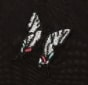••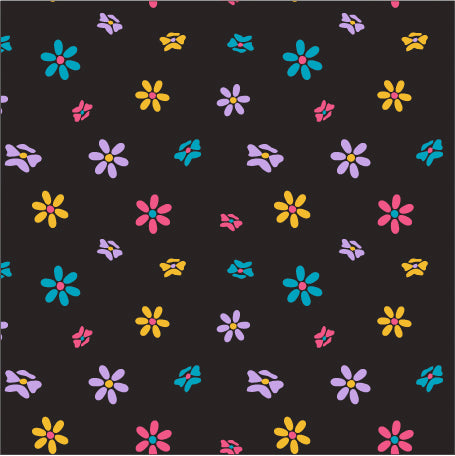•• XS
• XS
• XS
• XS
• XS
• XS
• XS
• XS
• S
• S
• S
• S
• S
• S
• S
• S
• M
• M
• M
• M
• M
• M
• M
• M
• L
• L
• L
• L
• L
• L
• L
• L
• XL
• XL
• XL
• XL
• XL
• XL
• XL
• XL
• 2XL
• 2XL
• 2XL
• 2XL
• 2XL
• 2XL
• 2XL
• 2XL
• 3XL
• 3XL
• 3XL
• 3XL
• 3XL
• 3XL
• 3XL
• 3XL
• 1+
• 1+
• 1+
• 1+
• 1+
• 1+
• 1+
• 1+
• 2+
• 2+
• 2+
• 2+
• 2+
• 2+
• 2+
• 2+
• 3+
• 3+
• 3+
• 3+
• 3+
• 3+
• 3+
• 3+

Lush Rib

17

### Triangle Bralette

\$48

\$33.60

••••••••• XS
• XS
• XS
• XS
• XS
• XS
• XS
• XS
• XS
• XS
• S
• S
• S
• S
• S
• S
• S
• S
• S
• S
• M
• M
• M
• M
• M
• M
• M
• M
• M
• M
• L
• L
• L
• L
• L
• L
• L
• L
• L
• L
• XL
• XL
• XL
• XL
• XL
• XL
• XL
• XL
• XL
• XL
• 2XL
• 2XL
• 2XL
• 2XL
• 2XL
• 2XL
• 2XL
• 2XL
• 2XL
• 2XL
• 3XL
• 3XL
• 3XL
• 3XL
• 3XL
• 3XL
• 3XL
• 3XL
• 3XL
• 3XL
• 1+
• 1+
• 1+
• 1+
• 1+
• 1+
• 1+
• 1+
• 1+
• 1+
• 2+
• 2+
• 2+
• 2+
• 2+
• 2+
• 2+
• 2+
• 2+
• 2+
• 3+
• 3+
• 3+
• 3+
• 3+
• 3+
• 3+
• 3+
• 3+
• 3+

Sexy Silky Mesh

37

### Strappy Triangle Bralette

\$51–\$57

\$35.70–\$39.90

•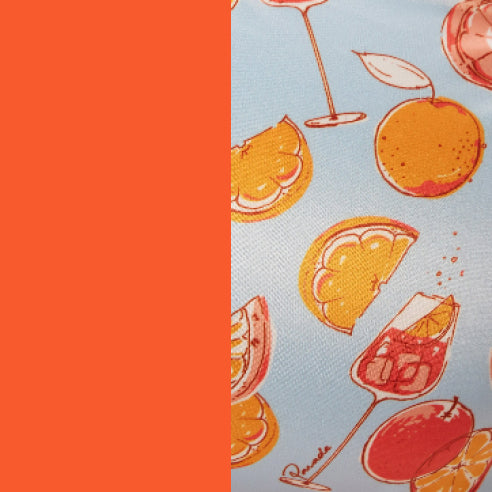••••••••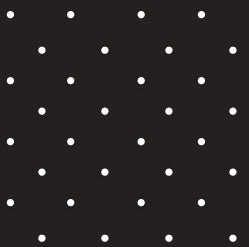•• XS
• XS
• XS
• XS
• XS
• XS
• XS
• XS
• XS
• XS
• S
• S
• S
• S
• S
• S
• S
• S
• S
• S
• M
• M
• M
• M
• M
• M
• M
• M
• M
• M
• L
• L
• L
• L
• L
• L
• L
• L
• L
• L
• XL
• XL
• XL
• XL
• XL
• XL
• XL
• XL
• XL
• XL
• 2XL
• 2XL
• 2XL
• 2XL
• 2XL
• 2XL
• 2XL
• 2XL
• 2XL
• 2XL
• 3XL
• 3XL
• 3XL
• 3XL
• 3XL
• 3XL
• 3XL
• 3XL
• 3XL
• 3XL
• 1+
• 1+
• 1+
• 1+
• 1+
• 1+
• 1+
• 1+
• 1+
• 1+
• 2+
• 2+
• 2+
• 2+
• 2+
• 2+
• 2+
• 2+
• 2+
• 2+
• 3+
• 3+
• 3+
• 3+
• 3+
• 3+
• 3+
• 3+
• 3+
• 3+

SuperSoft

34

### Strappy Bralette

\$48–\$51

\$33.60–\$35.70

••••••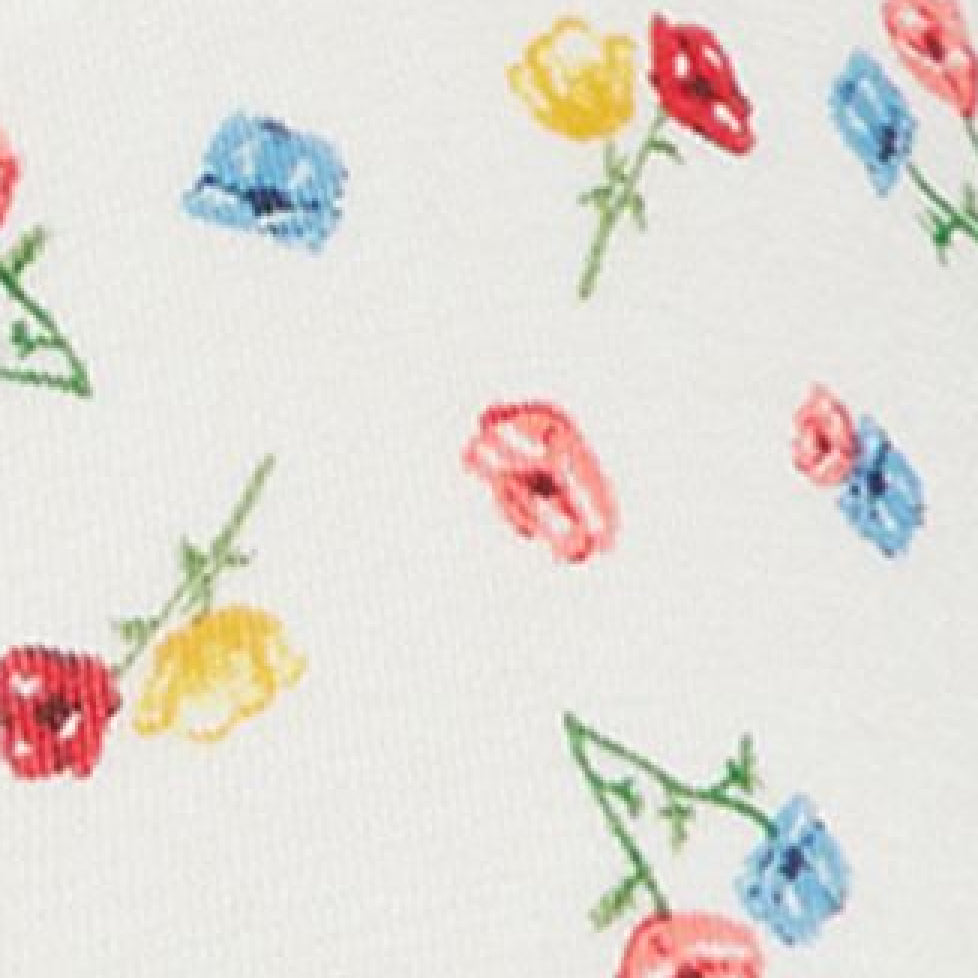••••• XS
• XS
• XS
• XS
• XS
• S
• S
• S
• S
• S
• M
• M
• M
• M
• M
• L
• L
• L
• L
• L
• XL
• XL
• XL
• XL
• XL
• 2XL
• 2XL
• 2XL
• 2XL
• 2XL
• 3XL
• 3XL
• 3XL
• 3XL
• 3XL
• 1+
• 1+
• 1+
• 1+
• 1+
• 2+
• 2+
• 2+
• 2+
• 2+
• 3+
• 3+
• 3+
• 3+
• 3+

Ultra-Soft Re:Play Naked

21

### Triangle Bralette

\$42

\$29.40

•••••• XS
• XS
• XS
• S
• S
• S
• M
• M
• M
• L
• L
• L
• XL
• XL
• XL
• 2XL
• 2XL
• 2XL
• 3XL
• 3XL
• 3XL
• 1+
• 1+
• 1+
• 2+
• 2+
• 2+
• 3+
• 3+
• 3+

Sexy Silky Mesh

40

### Triangle Bralette Hardware

\$48

\$33.60

•••• New!
• XS
• XS
• XS
• XS
• XS
• S
• S
• S
• S
• S
• M
• M
• M
• M
• M
• L
• L
• L
• L
• L
• XL
• XL
• XL
• XL
• XL
• 2XL
• 2XL
• 2XL
• 2XL
• 2XL
• 3XL
• 3XL
• 3XL
• 3XL
• 3XL

Sexy Silky Mesh

### Luxe Triangle Bodysuit

\$51

\$35.70

•••••• ### Re:Play Triangle Bralette

“This bralette is so comfy and sexy I did not want to take it off.”

Muriel W.